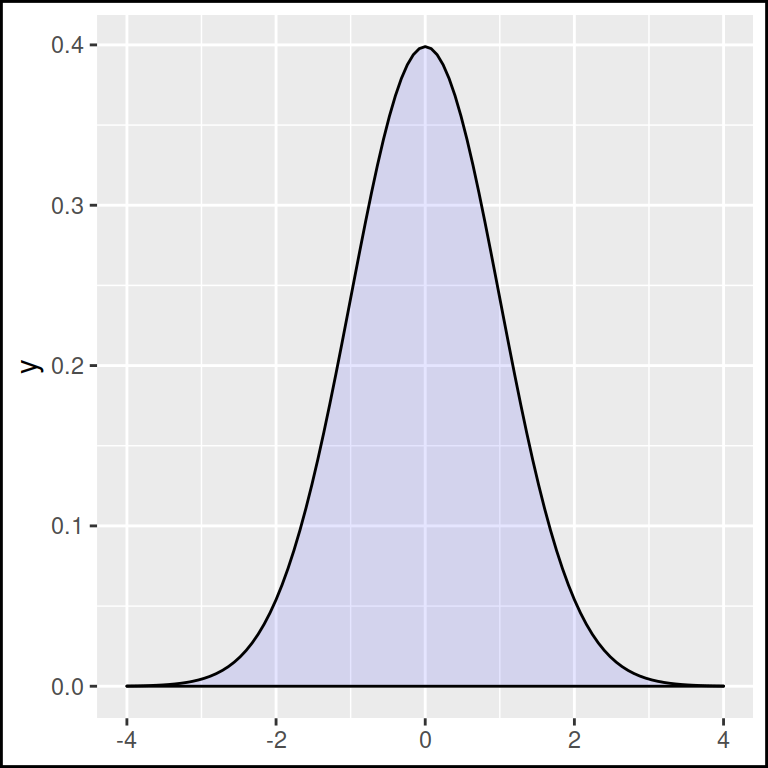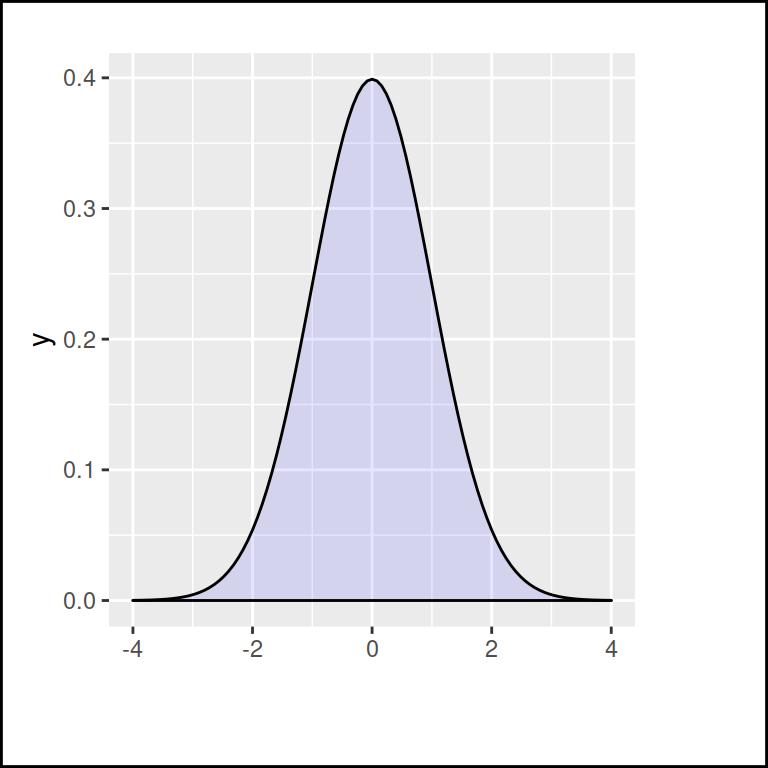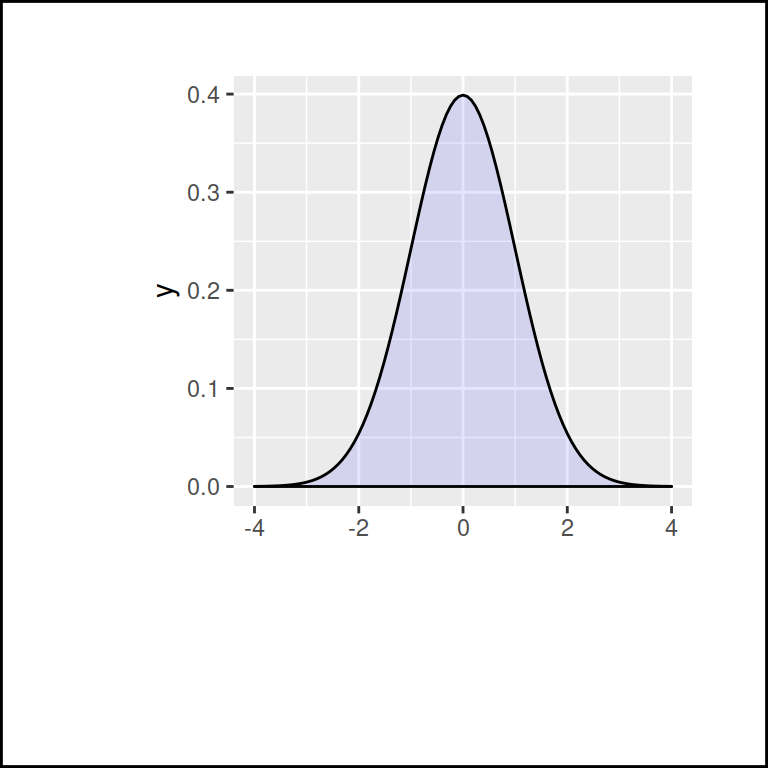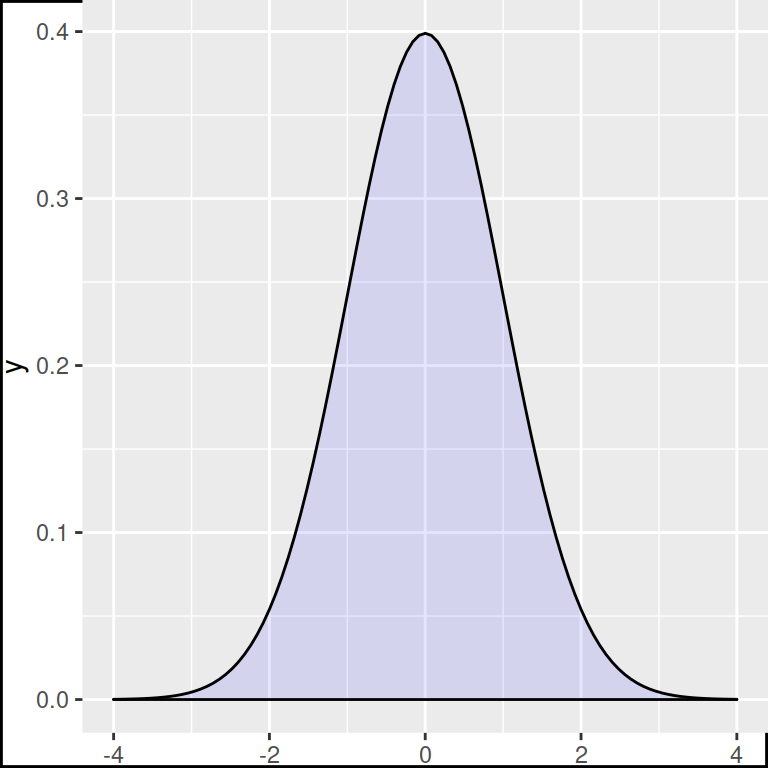# Margins in ggplot2

The margins of the plots made with ggplot2 will adjust automatically to new layers, e.g. if you add a title. We have added a black box around the sample plot so you can see how margins change.

``````library(ggplot2)

ggplot() +
stat_function(fun = dnorm, geom = "density",
xlim = c(-4, 4),
fill = rgb(0, 0, 1, 0.1)) +
theme(plot.background = element_rect(color = 1,
size = 1))``````## Increase marginsIn order to modify the plot margins set the `margin` function inside the `plot.margin` component of the `theme` function.

``````library(ggplot2)

ggplot() +
stat_function(fun = dnorm, geom = "density",
xlim = c(-4, 4),
fill = rgb(0, 0, 1, 0.1)) +
theme(plot.background = element_rect(color = 1,
size = 1),
plot.margin = margin(t = 20,  # Top margin
r = 50,  # Right margin
b = 40,  # Bottom margin
l = 10)) # Left margin``````The margins are measured with points (`"pt"`), but you can use other unit measure in the `unit` argument, like centimeters. Type `?unit` to see all the possible measures.

``````library(ggplot2)

ggplot() +
stat_function(fun = dnorm, geom = "density",
xlim = c(-4, 4),
fill = rgb(0, 0, 1, 0.1)) +
theme(plot.background = element_rect(color = 1,
size = 1),
plot.margin = margin(t = 1,  # Top margin
r = 1,  # Right margin
b = 3,  # Bottom margin
l = 2,  # Left margin
unit = "cm")) ``````

## Remove margins

To remove the margins set all values to 0. Note that there is still space to fit all the elements of the plot. You can set negative values to reduce more the margins.

``````library(ggplot2)

ggplot() +
stat_function(fun = dnorm, geom = "density",
xlim = c(-4, 4),
fill = rgb(0, 0, 1, 0.1)) +
theme(plot.background = element_rect(color = 1,
size = 1),
plot.margin = margin(t = 0,  # Top margin
r = 0,  # Right margin
b = 0,  # Bottom margin
l = 0)) # Left margin``````You can remember the order of the arguments of the `margin` function (t, r, b, l) remembering the word `tr`ou`bl`e.